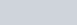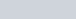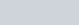Fill in the blanks to make each of the following a true statement:
Question:

Fill in the blanks to make each of the following a true statement:

(i)(ii) Φ′ ∩ A = …

(iii)(iv)Solution:

(i)(ii) Φ′ ∩ A = U ∩ A = A

∴ Φ′ ∩ A = A

(iii) A ∩ A′ = Φ

(iv) U′ ∩ A = Φ ∩ A = Φ

∴ U′ ∩ A = Φ